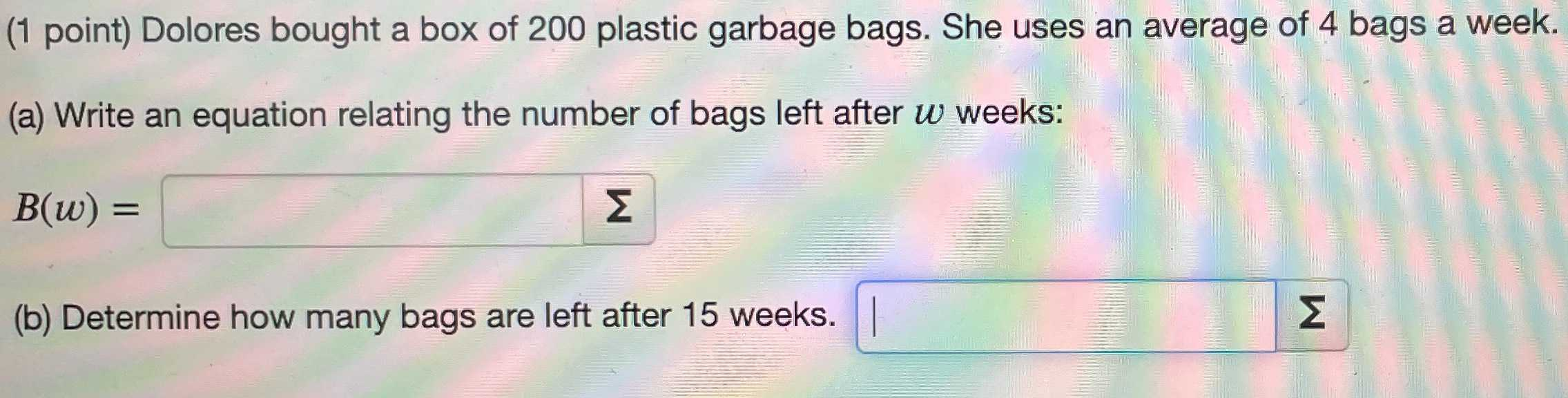### ¿Todavía tienes preguntas de matemáticas?

Pregunte a nuestros tutores expertos
Algebra
Pregunta(1 point) Dolores bought a box of $$200$$ plastic garbage bags. She uses an average of $$4$$ bags a week. (a) Write an equation relating the number of bags left after $$w$$ weeks:

$$B ( w ) = \square$$

(b) Determine how many bags are left after $$15$$ weeks. $$\square$$

$$B ( w ) = 200 - 4 w$$
$$140$$